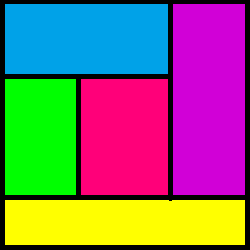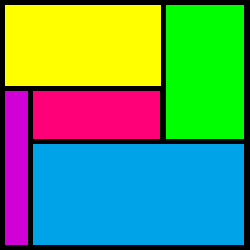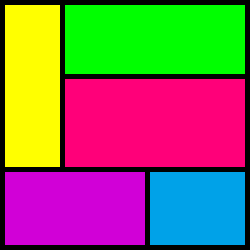#### You may also like### Consecutive Numbers

An investigation involving adding and subtracting sets of consecutive numbers. Lots to find out, lots to explore.### Calendar Capers

Choose any three by three square of dates on a calendar page...### Building Tetrahedra

Can you make a tetrahedron whose faces all have the same perimeter?

# 11x11 Square

##### Age 11 to 16Challenge Level

Start by asking students to consider which of the three diagrams could be a possible solution, and discuss the reasons why the other two are not possible.Some possible questions are:

• In the first diagram, what can you say about the yellow rectangle (the one at the bottom)?
• In the first diagram, what do the red and green rectangles have in common?

Ask students to draw possible arrangements of five rectangles that avoid any two of them having the same height or width.

Some further questions:

• Is it possible to have a rectangle with length $9$ in the centre of the diagram?
• What about one with length $8$?
• How many ways can you write $11$ as the sum of two positive integers?
• What must the areas of the five rectangles add up to?

This problem featured in the NRICH Secondary webinar in April 2022.